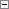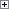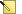A conditional statement is one type of control structure in C/AL.

You use conditional statements to specify a condition and one or more commands to execute if the condition is evaluated as true or false. There are two types of conditional statements in C/AL:

• IF-THEN-ELSE, where there are two choices.
• CASE, where there are more than two choices.

IF-THEN ELSE Statements

IF-THEN-ELSE statements have the following syntax.Copy Code
IF <Condition> THEN
<Statement1>
[ELSE
<Statement2>]

If <Condition> is true, then <Statement1> is executed. If <Condition> is false, then <Statement2> is executed.

The square brackets around ELSE <Statement2> mean that this part of the statement is optional. The ELSE statement is used when different actions are executed depending on how <Condition> is evaluated.

You can build more complex control structures by nesting IF-THEN-ELSE statements. The following example is a typical IF-THEN-ELSE statement.Copy Code
IF <Condition1> THEN
IF <Condition2> THEN
<Statement1>
ELSE
<Statement2>

If <Condition1> is false, then nothing is executed. If <Condition1> and <Condition2> are both true, then <Statement1> is executed. If <Condition1> is true and <Condition2> is false, then <Statement2> is executed.Note
A semicolon in front of an ELSE statement is not allowed.

Reading several nested IF-THEN-ELSE statements can be very confusing but generally, an ELSE statement belongs to the last IF statement that lacks an ELSE statement.

Programming Conventions

IF and THEN should be on the same line. ELSE should be on a separate line.

If there are many or long expressions, THEN should be on a new line and be aligned with IF.

When you write IF expressions with THEN and ELSE parts, write them so that the THEN result is more probable than the ELSE one.

If the last statement in the THEN part of an IF-THEN-ELSE statement is an EXIT or an ERROR, do not continue with an ELSE statement.

ExampleCopy Code
IF x = y THEN
x := x + 1
ELSE
x := -x - 1;

ExampleCopy Code
IF (xxxxxxxxxx = yyy) AND
(aaa = bbbbbbbbbb)
THEN
x := a
ELSE
y := b;

ExampleCopy Code
IF x <> y THEN
EXIT(TRUE);
x := x * 2;
y := y - 1;

Incorrect ExampleCopy Code
IF x < y THEN
EXIT(TRUE)
ELSE BEGIN
x := x * 2;
y := y - 1;
END;

Example

The following example shows an IF-THEN statement without the optional ELSE statement.Copy Code
IF Amount < 1000 THEN
Total := Total + Amount;

Example

The following example shows a nested IF-THEN-ELSE statement.Copy Code
...
IF Amount < 1000 THEN BEGIN
IF I > J THEN
Max := I
ELSE
Max := J;
Amount := Amount * Max;
END
ELSE
...

CASE Statements

CASE statements have the following syntax.Copy Code
CASE <Expression> OF
<Value set 1>:
<Statement 1>;
<Value set 2>:
<Statement 2>;
...
...
<Value set n>:
<Statement n>;
[ELSE
<Statement n+1>]
END;

In this definition, <Expression> cannot be a record and <Value set> must be an expression or a range.

CASE statements are also called multiple option statements and are typically used when you must choose between more than two different actions. The function of the CASE statement is as follows:

• The <Expression> is evaluated, and the first matching value set executes the associated statement, if there is one.
• If no value set matches the value of the expression and the optional ELSE part has been omitted, then no action is taken. If the optional ELSE part is used, then the associated statement is executed.

The data type of the value sets must be the same as the data type of <Expression> or at least be convertible to the same data type.

In most cases, the data type of the value sets are converted to the date type of the evaluated expression. The only exception is if the evaluated expression is a Code variable. If the evaluated expression is a Code variable, then the value sets are not converted to the Code data type.Note
This type conversion can cause an overflow at run time if the resulting data type cannot hold the values of the datasets.

For more information about Code variables, see Code Data Type.

Programming Conventions

When you use a CASE statement, indent the value sets by two character spaces. If you have two or more value sets on the same line, then separate them by commas without spaces. The last value set on a line is immediately followed by a colon without a preceding space. The action starts on the line after the value set and is further indented by two character spaces. If there is a BEGIN, then it should be put on a separate line unless it follows ELSE. If a BEGIN follows an ELSE, then it should be on the same line as ELSE.

If there are more than two alternatives, use a CASE statement. Otherwise, use an IF-THEN-ELSE statement.

ExampleCopy Code
CASE Field OF
Field::A:
BEGIN
x := x + 1;
y := -y - 1;
END;
Field::B:
x := y;
Field::C,Field::D:
y := x;
ELSE BEGIN
y := x;
a := b;
END;
END

Example

The following C/AL code prints various messages depending on the value of Number. If the value of Number does not match any of the entries in the CASE structure, then the ELSE entry is used as the default.Copy Code
CASE Number OF
1,2,9:
MESSAGE('1, 2, or 9.');
10..100:
MESSAGE('In the range from 10 to 100.');
ELSE
MESSAGE('Neither 1, 2, 9, nor in the range from 10 to 100.');
END

Example

The following C/AL code shows how value sets in a CASE statement are evaluated if the expression is a Code data type. This example requires that you create the following variable.

Variable Data type

MyCode

CodeCopy Code
MyCode := 'ABC';
CASE MyCode OF
'abc':
MESSAGE('This message is not displayed.');
'def':
MESSAGE('This message is not displayed.);
ELSE
MESSAGE('The value set does not match the expression.');

The value set 'abc' is not converted because the evaluated expression MyCode is a Code variable.